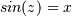# numpy.arcsin¶

`numpy.``arcsin`(x, /, out=None, *, where=True, casting='same_kind', order='K', dtype=None, subok=True[, signature, extobj]) = <ufunc 'arcsin'>

Inverse sine, element-wise.

Parameters: x : array_like y-coordinate on the unit circle. out : ndarray, None, or tuple of ndarray and None, optional A location into which the result is stored. If provided, it must have a shape that the inputs broadcast to. If not provided or None, a freshly-allocated array is returned. A tuple (possible only as a keyword argument) must have length equal to the number of outputs. where : array_like, optional Values of True indicate to calculate the ufunc at that position, values of False indicate to leave the value in the output alone. **kwargs For other keyword-only arguments, see the ufunc docs. angle : ndarray The inverse sine of each element in x, in radians and in the closed interval `[-pi/2, pi/2]`. If x is a scalar, a scalar is returned, otherwise an array.

Notes

`arcsin` is a multivalued function: for each x there are infinitely many numbers z such that. The convention is to return the angle z whose real part lies in [-pi/2, pi/2].

For real-valued input data types, arcsin always returns real output. For each value that cannot be expressed as a real number or infinity, it yields `nan` and sets the invalid floating point error flag.

For complex-valued input, `arcsin` is a complex analytic function that has, by convention, the branch cuts [-inf, -1] and [1, inf] and is continuous from above on the former and from below on the latter.

The inverse sine is also known as asin or sin^{-1}.

References

Abramowitz, M. and Stegun, I. A., Handbook of Mathematical Functions, 10th printing, New York: Dover, 1964, pp. 79ff. http://www.math.sfu.ca/~cbm/aands/

Examples

```>>> np.arcsin(1)     # pi/2
1.5707963267948966
>>> np.arcsin(-1)    # -pi/2
-1.5707963267948966
>>> np.arcsin(0)
0.0
```

numpy.tan

numpy.arccos